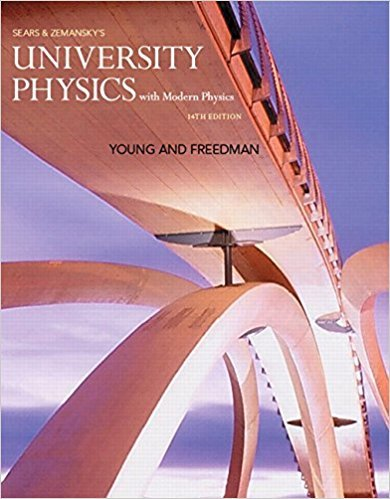×
Log in to StudySoup
Get Full Access to University Physics With Modern Physics (1) - 14 Edition - Chapter 34 - Problem Q34.4
Join StudySoup for FREE
Get Full Access to University Physics With Modern Physics (1) - 14 Edition - Chapter 34 - Problem Q34.4

Already have an account? Login here
×
Reset your password

# Solved: Explain why the focal length of a plane mirror isISBN: 9780321973610 228

## Solution for problem Q34.4 Chapter 34

University Physics with Modern Physics (1) | 14th Edition

• Textbook Solutions
• 2901 Step-by-step solutions solved by professors and subject experts
• Get 24/7 help from StudySoup virtual teaching assistantsUniversity Physics with Modern Physics (1) | 14th Edition

4 5 1 405 Reviews
26
1
Problem Q34.4

Explain why the focal length of a plane mirror is infinite, and explain what it means for the focal point to be at infinity.

Step-by-Step Solution:
Step 1 of 3

Faraday’s Law of Induction  Potential drop along the closed contour is minus the rate of change of magnetic flux.  We can change the magnetic flux in several ways including changing the magnitude of the magnetic field, changing the area of the loop, or by changing the angle the loop with respect to the magnetic field 10 Lenz’s Law (1)  Lenz’s Law defines a rule for determining the direction of an induced current in a loop  An induced current will have a direction such that the magnetic field due to the induced current opposes the change in the magnetic flux that induces the current  Meaning, if the flux increases, the induced B-field will be direc

Step 2 of 3

Step 3 of 3

##### ISBN: 9780321973610

University Physics with Modern Physics (1) was written by and is associated to the ISBN: 9780321973610. The answer to “Explain why the focal length of a plane mirror is infinite, and explain what it means for the focal point to be at infinity.” is broken down into a number of easy to follow steps, and 24 words. This full solution covers the following key subjects: . This expansive textbook survival guide covers 44 chapters, and 4574 solutions. This textbook survival guide was created for the textbook: University Physics with Modern Physics (1), edition: 14. Since the solution to Q34.4 from 34 chapter was answered, more than 244 students have viewed the full step-by-step answer. The full step-by-step solution to problem: Q34.4 from chapter: 34 was answered by , our top Physics solution expert on 01/09/18, 07:46PM.

Unlock Textbook Solution

Enter your email below to unlock your verified solution to:

Solved: Explain why the focal length of a plane mirror is